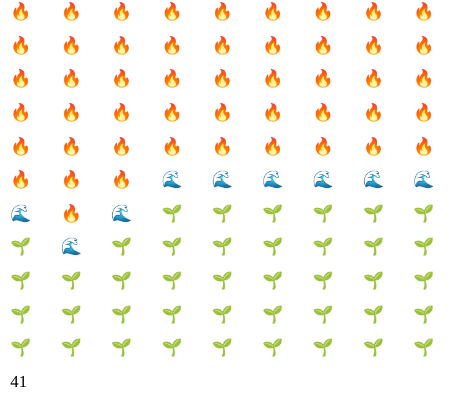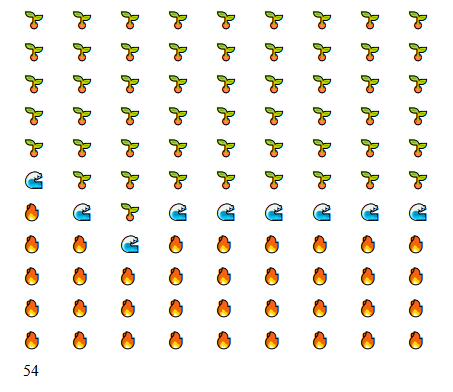Flood plants by clicking on them to stop the spread of flames. Save as many plants as possible!

This game was written in 498 characters for the Eggplant Show Community Jam #3 in April 2020. The theme of this jam was "Keeping it Short".

I decided to apply this theme to the source code of my entry in the spirit of TweetTweetJam. Create a game that fits inside the length of two tweets (560 characters).

You can mess with the JavaScript source on Codepen.

```d=document
p=a=>{e=d.createElement('p');a.append(e);return e;}
y=p(d.body)
s=y.style
s.display='grid'
s.gridTemplate=`\${'2em '.repeat(11)}/\${'3em '.repeat(9)}`
n='innerText'
o='🌱';r='🔥'
h=Array(99).fill(o)
g=h.map(a=>p(y))
m=a=>{h.map((v,i)=>g[i][n]=v);z[n]=h.reduce((e,v)=>v==o?e+1:e,0)}
z=p(y)
u=a=>h.map((e,i)=>e!=o?e:h[i+1]==r&&i%9!=8||h[i-1]==r&&i%9!=0||h[i+9]==r||h[i-9]==r?r:e)
g.map((e,i)=>e.onclick=a=>{e[n]==o&&(h[i]='🌊');h=u();m()})
q=a=>Math.random()*99|0
h[q()]=r;h[q()]=r;h[q()]=r
m()
```
 Status Released Platforms HTML5 Author Ryan Kubik Genre Puzzle, Simulation Tags Turn-based

great game